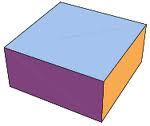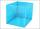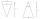# Rectangular cuboid

The rectangular cuboid has a surface area 5334 cm2, and its dimensions are in the ratio 2:4:5. Find the volume of this rectangular cuboid.

V =  23519.0145 cm3

### Step-by-step explanation:Did you find an error or inaccuracy? Feel free to write us. Thank you!Amy
Not very useful plz give worked example with steps

4 years ago  2 LikesDr Math
Example is complete with solution  step-by-stepTips to related online calculators
Looking for help with calculating roots of a quadratic equation?
Check out our ratio calculator.
Do you have a linear equation or system of equations and looking for its solution? Or do you have a quadratic equation?
Tip: Our volume units converter will help you with the conversion of volume units.

## Related math problems and questions:

• Surface of cuboidFind the surface of the cuboid if its volume is 52.8 cm3 and the length of its two edges is 2 cm and 6 cm.
• Cuboid - ratiosThe sizes of the edges of the cuboid are in the ratio 2: 3: 5. The smallest wall has an area of 54 cm2. Calculate the surface area and volume of this cuboid.
• Two rectangular boxesTwo rectangular boxes with dimensions of 5 cm, 8 cm, 10 cm, and 5 cm, 12 cm, 1 dm are to be replaced by a single cube box of the same cubic volume. Calculate its surface.
• Cuboid to cubeA cuboid with dimensions of 9 cm, 6 cm, and 4 cm has the same volume as a cube. Calculate the surface of this cube.
• Cuboid surfaceDetermine surface area of cuboid if its volume is 52.8 cm cubic and length of the two edges are 2 cm and 6 cm.
• Cuboid - edgesThe cuboid has dimensions in ratio 4: 3: 5, the shortest edge is 12 cm long. Find: (A) the lengths of the remaining edges, (B) the surface of the cuboid, (C) the volume of the cuboid
• The cubeThe cube has a surface of 600 cm2. What is its volume?
• The cubeThe cube has a surface area of 486 m ^ 2. Calculate its volume.
• Area to volumeIf the surface area of a cube is 486, find its volume.
• CuboidCuboid has a surface of 516 cm2. Side a = 6 cm and b = 12 cm. How long is the side c =?
• TrapezoidArea of trapezoid is 135 cm2. Sides a, c and height h are in a ratio 6:4:3. How long are a,c and h? Make calculation...
• Volume and surface areaFind the volume and surface of a wooden block with dimensions: a = 8 cm, b = 10 cm, c = 16 cm.
• Cube 5The surface of the cube is 15.36 dm2. How will change the surface area of this cube if the length of the edges is reduced by 2 cm?
• Body diagonalCalculate the volume of a cuboid whose body diagonal u is equal to 6.1 cm. Rectangular base has dimensions of 3.2 cm and 2.4 cm
• Largest possible coneIt is necessary to make the largest possible cone from an iron rod in the shape of a prism with dimensions of 5.6 cm, 4.8 cm, 7.2 cm. a) Calculate its volume. b) Calculate the waste.
• Cube surface and volumeFind the surface of the cube with a volume of 27 dm3.
• Cuboid and ratioFind the dimensions of a cuboid having a volume of 810 cm3 if the lengths of its edges coming from the same vertex are in ratio 2: 3: 5